Practicing OP Malhotra Class 9 Maths Solutions Chapter 8 Triangles Ex 8(B) is the ultimate need for students who intend to score good marks in examinations.

## S Chand Class 9 ICSE Maths Solutions Chapter 8 Triangles Ex 8(B)

Question 1.
The vertical angle of an isosceles triangle is 90°. Find each of its base angles.
Solution:
Sum of angles of a triangle = 180°
Vertical angle of an isosceles triangle = 90°
∴ Sum of its base angles = 180° – 90° = 90°
∵ Base angles are equal to each other
∴ Each angle will be = $$\frac { 90° }{ 2 }$$ = 45°
Hence each base angle = 45°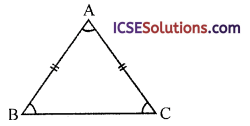Question 2.
Prove that each angle of an equilateral triangle is 60°. Hence show that every equilateral triangle is equiangular. What is the measure of each of the exterior angle of an equilateral triangle ?
Solution:
Given : An equilateral ∆ABCTo Prove:
(i) Each angle is of 60°
(ii) What is its each exterior angle.
Proof: In ∆ABC,
∵ AB = AC (sides of equilateral triangle)
∴ ∠C = ∠B (angles opposite to equal sides) … (i)
Similarly, AC = CB
∴ ∠B = ∠A … (ii)
From (i) and (ii)
∠A = ∠B = ∠C
But ∠A + ∠B + ∠C = 180°
(sum of angles of a triangle)
∴ ∠A = ∠B = ∠C = $$\frac { 180° }{ 3 }$$ = 60°
Hence proved.
∵ Each equilateral triangle has 60° each
∴ Every equilateral triangle is equiangular
∵ ∠ABC = 60°
and ∠ABC + ∠CBZ = 180° (linear pair)
⇒ 60° + ∠CBZ = 180° ⇒ ∠CBZ =180°- 60° = 120°
∴ Each exterior angle of an equilateral triangle measures 120°.Question 3.
Prove that if the base of an isosceles triangle is produced at both ends, the exterior angles so formed are equal to each other?
Solution:
Given : ABC is an isosceles triangle in which AB = AC
BC is produced to both sides to D and E forming exterior angles ACD and ABE respectively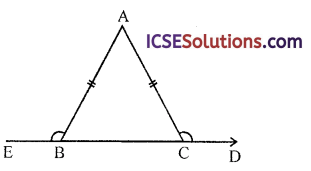To prove : ∠ACD = ∠ABE
Proof: In ∆ABC,
∵ AB = AC (given)
∴ ∠C = ∠B (angles opposite to equal sides)
or ∠ACB = ∠ABC
But ∠ABE + ∠ABC = 180° (linear pair) … (i)
Similarly ∠ACD + ∠ACB = 180° … (ii)
From (i) and (ii)
∠ABE + ∠ABC = ∠ACD + ∠ACB
But ∠ABC = ∠ACB (proved)
∠ABE = ∠ACD or ∠ACD = ∠ABE
Hence proved.

Question 4.
In a ∆ABC, AD bisects ∠BAC and AD = DC. If ∠BDA = 70°, calculate ∠ACD and ∠ABD.
Solution:
In ∆ABC, AD is the bisector of ∠BAC which meets BC at AD, AD = DC and ∠BDA = 70°
∵ AD is the bisector of ∠BAC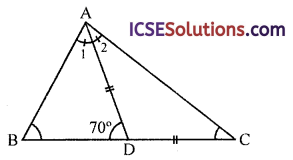∴ ∠1 = ∠2
∴ ∠2 = ∠C
But Ext. ∠ADB = ∠2 + ∠C
⇒ 70° = ∠2 + ∠C = ∠C + ∠C = 2 ∠C
∴ ∠C = $$\frac { 70° }{ 2 }$$ = 35°
In ∆ABD,
∠1 + ∠B + ∠ADB = 180°
(sum of angles of a triangle)
⇒ ∠2 + ∠B + 70° = 180° (∵ ∠1 = ∠2 = ∠C)
⇒ ∠C + ∠B + 70° = 180°
⇒ 35° + ∠B + 70° = 180°
⇒ ∠B + 105° = 180°
⇒ B = 180° – 105° = 75°
Hence ∠ACD = 35° and ∠ABD = 75°

Question 5.
What are the measures of the angles of an isosceles triangle in which each of the base angle is:
(i) Double the vertical angle ?
(ii) Thrice the vertical angle ?
Solution:
(i) Let the vertical angle of an isosceles triangle = x
Then each equal angle = 2x
∴ x + 2x + 2x = 180°
(sum of angles of a triangle)
⇒ 5x = 180°
⇒ x = $$\frac { 180° }{ 5 }$$ = 36°
∴ Vertical angle = 36°
and each of base anlges = 36° x² = 12°
Hence angles are 36°, 12°, 12°

(ii) Let vertical angle of an isosceles triangle = x
Then each base angle = 3x
∴ x + 3x + 3x = 180°
(sum of angles of a triangle)
⇒ 7x = 180°
⇒ x = $$\frac { 180° }{ 7 }$$
∴ Vertical angle = $$\frac { 180° }{ 7 }$$ = 25 $$\frac { 5° }{ 7 }$$
and each base angle = $$\frac { 180° }{ 7 }$$ x 3 = $$\frac { 540° }{ 7 }$$
= 77$$\frac { 1° }{ 7 }$$
∴ Angle are 25$$\frac { 5° }{ 7 }$$ , 77 $$\frac { 1° }{ 7 }$$ and 77 $$\frac { 1° }{ 7 }$$

Question 6.
Find the lettered angles in each of the following figures :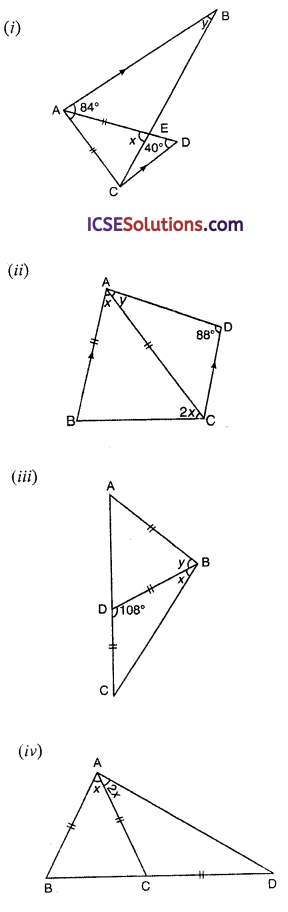Solution:
(i) ∵ AB || CD and BC is its transversal
∴ ∠y = ∠1 (alternate angle)
In ∆ECD,
Ext. ∠x = ∠C + ∠D = ∠1 + 40°
In ∆ACE,
AE = AC (given)
∴ ∠ACE = ∠AEC = x
∵ AB || CD
∴ ∠BAC + ∠ACB = 180° (colinear angles)
84° + x + ∠1 = 180°
⇒ x + ∠L = 180° – 84° = 96°
⇒ ∠1 + 40° + ∠L = 96° (∵ x = ∠1 + 40°)
⇒ 2 ∠1 = 96 – 40 = 56
⇒ ∠1 = $$\frac { 56° }{ 2 }$$ = 28°
⇒ ∠y = 28° (∵ ∠1 = ∠y)
But x = ∠1 + 40° = 28° + 40° = 68°
Hence x = 68°, y = 28°(ii) In the figure in ∆ABC,
AB = AC
∴ ∠B = ∠ACB = 2x
But ∠BAC + ∠ABC + ∠ACB = 180° (angles of a triangle)
⇒ x + 2x + 2x = 180°
⇒ 5x = 180°
x = $$\frac { 180° }{ 5 }$$ = 36°
∵ AB || CD
∴ ∠ACD = ∠BAC (alternate angles)
= x = 36°
Now in ∆ACD,
⇒ y + x + 88° = 180° ⇒ y + 36° + 88° = 180°
⇒ y + 124° = 180° ⇒ y = 180° – 124° = 56°
Hence x = 36°, y = 56°

(iii) In the figure,
AB = BD = DC
∠BDC = 108°
In ∆BCD,
∵ BD = DC (given)
∴ ∠DCB = ∠DBC = x
But ∠DCB + ∠DBC + ∠BDC = 180°
⇒ x + x + 108° = 180°
⇒ 2x = 180°- 108° = 72°
∴ x = $$\frac { 72° }{ 2 }$$ = 36°
In ∆ABD,
BA = BD (given)
(angles opposite to equal sides)
But ∠BDA = 180° – 108° = 72°
(∵ ∠BDC + BDA = 180°)
(linear pair)
∴ ∠BAD = ∠BDA = 72°
and Ext. ∠BDC = ∠BAD + ∠ABD
⇒ 108° = 72° + y²
⇒ y = 108°- 72° = 36°
Hence x = 36° and y = 36°

(iv) In ∆ABC, AB = AC
and BC is produced to D
Such that AC = CD
In ∆ACD,
∵ AC = CD (given)
∴ ∠CAD = ∠CDA = 2x
and Ext. ∠ACB = ∠CAD + ∠CDA = 2x + 2x = 4x
∵ In AABC, AB = AC
∴ ∠ABC = ∠ACB = 4x
∴ But ∠BAC +∠ABC + ∠ACB = 180° (angles of a triangle)
∴ 4x + 4x + x = 180° ⇒ 9x = 180°
⇒ x = $$\frac { 180° }{ 2 }$$ = 20°
Hence x = 20°

Question 7.
In figure, LM = LN, ∠FLN = 110°.
Calculate:
(i) ∠LMN
(ii) ∠MLN.Solution:
In the figure, LM = LN
∠FLN = 110°, ∠F = ∠Q = 90°
∠F + ∠Q + ∠QNL + ∠FLN = 360°
⇒ 90° + 90° + ∠QNL + 110° = 360°
⇒ 290° + ∠QNL = 360°
⇒ ∠QNL = 360° – 290° = 70°
In ∆LMN
∵ LM = LN
(i) ∴ ∠LMN = ∠LNM or ∠LNQ = 70°
(ii) ∠LMN = ∠MLN = 180°- ∠LMN + ∠LNM
= 180° – 70° – 70° = 180°- 140° = 40°
Hence ∠QNL = 70° and ∠MLN = 40°

Question 8.
In figure, O is the centre of the circular arc ABC. Find the angles of AABC.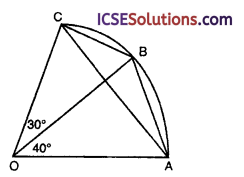Solution:
OABC is a sector
∠AOB = 40° and ∠BOC = 30°
AB, BC and CA are joined
∵ OA = OB = OC (radii of the arc ABC)
∴ In ∆AOB,
∠OAB = ∠OBA and ∠OBC = ∠OCB
(angles opposite to equal sides)
But ∠AOB = 40° and ∠BOC = 30°
∴ ∠OAB = ∠OBA = $$\frac{180^{\circ}-40^{\circ}}{2}=\frac{140^{\circ}}{2}$$ = 70°
and ∠OBC = ∠OCB = $$\frac{180^{\circ}-30^{\circ}}{2}=\frac{150^{\circ}}{2}$$ = 75°
∴ ∠ABC = 70° + 75° = 145°
Arc AB subtends ∠AOB at the centre and ∠ACB at the remaining part of the circle
∴ ∠ACB = $$\frac { 1 }{ 2 }$$ ∠AOB = $$\frac { 1 }{ 2 }$$ x 40° = 20°
Similarly ∠CAB = $$\frac { 1 }{ 2 }$$ ∠BOC = $$\frac { 1 }{ 2 }$$ x 30° = 15°
Hence angles of ∆ABC are 15°, 20° and 145°

Question 9.
In figure, ABC is an isosceles triangle with AB equal to AC. AC is produced to point D and CE is drawn parallel to BA. If ∠CBA = 52°, find ∠DCE.Solution:
In the figure, ∆ABC in which AB = AC, CE || M
and AC is produced to D
∠ABC = 52°
Now we have to find ∠DCE
In AABC,
AB =AC (given)
∠ACB = ∠ABC = 52°
(angles opposite to equal sides) AB || EC (given)
∠A = ∠ACE (alternate angles)
But ∠A = 180°- (∠ABC + ∠ACB)
= 180° – (52° + 52°) = 180° – 104° = 76° ∠ACE = ∠A = 76°
But ∠ACE + ∠ECD = 180° (linear pair) ⇒ 76° +∠ECD = 180°
⇒ ∠ECD = 180° – 76°= 104° or ∠DCE = 104°

Question 10.
In figure, it is given that AB = AC and DA is parallel to BC.
∠DAB = 70°. Find ∠BAC.Solution:
In the figure, in ∆ABC,
AB = AC and AD || BC is drawn
∠DAB = 70°,We have to find ∠BAC
∵ DA || BC
∴ ∠ABC = ∠DAB (alternate angles)
But in ∆ABC, AB = AC
∴ ∠ABC = ∠ACB
(angles opposite to equal sides)
∴ ∠ACB = ∠ABC = 70°
Now ∠BAC + ∠ABC + ∠ACB = 180° (angles of a triangle)
⇒ ∠BAC + 70° + 70° = 180°
⇒ ∠BAC + 140° = 180°
⇒ ∠BAC = 180° – 140° = 40°
Hence ∠BAC = 40°

Question 11.
In figure, angles ACB is a right angle, AC = CD, CDEF is a rectangle, and ∠BAC = 50°.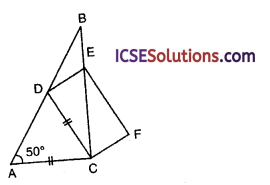(i angle BDE;
(ii) the angle between the diagonals CE, DF of the rectangle.
Solution:
∠ACB is a right angle
AC = CD, CDEF is a rectangle and ∠BAC = 50°Join DF
We have to find
(i) ∠BDE
(ii) the angle between the diagonals CE and DF of rectangle CDEF
In AADC, AC = CD (given)
∠CDA = ∠DAC or ∠BAC
(angles opposite to equal sides)
∠CDA = 50°

(i) Now ∠CDA + ∠CDE + ∠BDE = 180°
50°+ 90° +∠BDE = 180°
⇒ 140° +∠BDE = 180°
⇒ ∠BDE = 180° – 140°
⇒ ∠BDE = 40°

(ii) ∴ DE = EB
In ∆DAC,
∠ACD = 180° – (∠A + ∠ADC)
= 180° – (50° + 50°) = 180° – 100° = 80°
∴ ∠DCO = ∠ACB – ∠ACD = 90° – 80° = 10°
In ∆OCD,
∵ OC = OD
∴ ∠ODC = ∠OCD
∴ ∠COD = 180° – (10°+ 10°)
= 180° – 20° = 160°
Hence ∠BDE = 40° and ∠COD = 160°Question 12.
ABC is a triangle. The bisector of the angle BCA meets AB in X. A point Y lies on CX such that AX = AY. Prove that ∠CAY = ∠ABC. Solution:
Given : In ∆ABC, bisector of ∠BCA meets AB in X. A point Y lies on CX such that AX = AY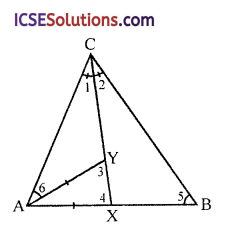To prove : ∠CAY = ∠ABC
Proof: In ∆ABC,
∵ CX is the bisector of ∠C
∴ ∠1 = ∠2
In ∆AXY,
∵ AX = AY
∴ ∠3 = ∠4
In ∆BCX
Ext. ∠4 = ∠2 + ∠5
= ∠1 + ∠5 … (i) (∵ ∠1 = ∠2)
Similarly in ∆CAY
Ext. ∠3 = ∠1 + ∠6 … (ii)
From (i) and (ii)
∠3 = ∠4
∴ ∠1 + ∠5 = ∠1 + ∠6
⇒ ∠5 = ∠6
⇒ ∠CAY = ∠ABC
Hence proved.

Question 13.
In figure, PS = PR, ∠TPS = ∠QPR. Prove that PT = PQ.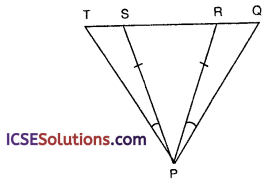Solution:
Given : In the figure,
PS = PR, ∠TPS = ∠QPR
To prove : PT = PQ
Proof: In ∆PRS,
∵ PS = PR (given)
∠PSR = ∠PRS
(angles opposite to equal sides)
But ∠PSR + ∠PST = 180° (linear pair)
Similarly ∠PRS + ∠PRQ = 180°
∠PSR + ∠PST = ∠PRS + ∠PRT
But ∠PSR = ∠PRS (proved)
∴ ∠PST = ∠PRQ
Now in APST and ∆PRQ,
PS = PR (given)
∠PST = ∠PRQ (proved)
∠TPS = ∠QPR (given)
∴ ∆PST ≅ ∆PRQ (ASA axiom)
∴ PT = PQ (c.p.c.t.)
Hence proved.

Question 14.
ABC is an isosceles triangle with AB = AC. BD and CE are two medians of the triangle. Prove that BD = CE.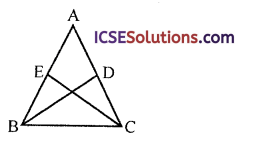Solution:
Given : In an isosceles ∆ABC, AB = AC
BD and CF are the medians of sides AC and AB respectively
To prove : BD = CE
Proof: In ∆BDC and ∆BEC
BC = BC (common)
∠C = ∠B
(base angles of an isosceles triangle)
CD = BE (half of equal sides)
∴ ∆BDC ≅ ∆BEC (SAS axiom)
∴ BD = CE (c.p.c.t.)
Hence proved.

Question 15.
Prove that the triangle formed by joining the midpoints of an isosceles triangles is also an isosceles triangle.
Solution:
Given : In an isosceles ∆ABC in which AB = AC.
D, E and F are the mid-points of the sides BC, CA and AB respectively
DE, EF and FD are joined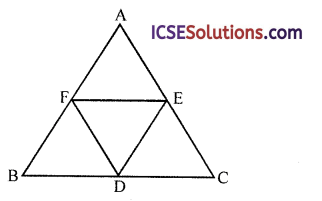To prove : ∆DEF is an isosceles triangle
Proof: ∵ D and F are the mid-points of BC and BA respectively
∴ DF || AC and $$\frac { 1 }{ 2 }$$ AC
Similarly D and E are the mid-points of BC and CA respectively
∴ DE || AB and $$\frac { 1 }{ 2 }$$ AB
But AB = AC (given)
∴ DE = DF
∴ ∆DEF is an isosceles triangle
Hence proved.

Question 16.
In the figure, AD = BC, AC = BD. Prove that PAB is an isosceles triangle.Solution:
Given : In the figure,
AD = BC, AC = BD
To prove : ∆PAB is an isosceles triangle
AB = AB (common)
BD = AC (given)
∴ ∆ADB ≅ ∆ACB (SSS axiom)
∴ ∠D = ∠C (c.p.c.t.)
Now in ∆APD and ∆BPC AD = BC (given)
∠APD = ∠BPC (vertically opposite angle) ∠D = ∠C (proved)
∴ ∆APD ≅ ∆BPC (AAS axiom)
∴ AP = BP (c.p.c.t.)
∴ ∆PAB is an isosceles triangle
Hence proved.

Question 17.
In the figure, (i) and (ii), AB = AC and BD = DC. Prove that ∠ABD = ∠ACD.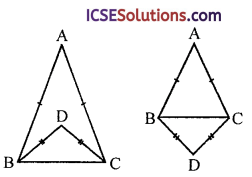Solution:
Given : In the figure (i) and (ii)
AB = AC, BD = DC
To prove : ∠ABD = ∠ACDProof:
∆ABD and ∆ACD
AB = AC (given)
DB = DC (given)
∴ ∆ABD ≅ ∆ACD (SSS axiom)
∴ ∠ABD = ∠ACD (c.p.c.t)
Hence proved.

Question 18.
In figure, ABC is an isosceles triangle in which AB = AC. The side BA is produced to D such that AB = AD. Prove that ∠BCD is a right angle.Solution:
Given : In ∆ABC, AB = AC
BA is produced to D
DC is joined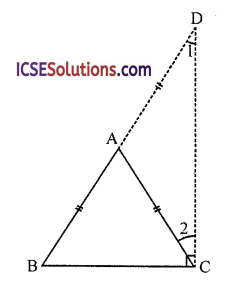To prove : ∠BCD = 1 right angle
Proof: In ∆ABC,
∵ AB = AC (given)
∴ ∠ABC = ∠ACB
(angles opposite to equal sides)
Similarly in ∆ACD
⇒ AC = AD (∵ AB = AC)
∴ ∠1 = ∠2
∠ABC + ∠1 = ∠ACB + ∠2
⇒ ∠ABC + ∠1 = ∠BCD
But ∠ABC + ∠1 + ∠BCD = 180°
(angles of a triangle)
∴ ∠BCD + ∠BCD = 180°
⇒ 2 ∠BCD = 180° ⇒ ∠BCD = $$\frac { 180° }{ 2 }$$ = 90°
∴ ∠BCD is a right angle
Hence proved.

Question 19.
In an isosceles triangle, prove that the bisectors of the base angles meeting the opposite sides are equal.
Solution:
Given : In ∆ABC, AB = AC
Bisectors of ∠B and ∠C meets AC and AB in D and E respectivelyTo prove : BD = CE
Proof: In ∆BDC and ∆BEC
BC = BC (common)
∠C = ∠B
(base angles of an isosceles triangle)
∠DBC = ∠ECB (half of equal angles)
∴ ∆BDC ≅ ∆BEC (ASA axiom)
∴ BD = CE (c.p.c.t.)

Question 20.
If the bisector of the vertical angle of a triangle bisects the base also, the triangle is isosceles.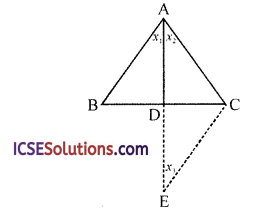Solution:
Given : In ∆ABC, the bisector of vertical angle A bisects the sides BC at D
∴ x1 = x2 and
BD = DC
To prove : AABC is an isosceles
i.e., AB = AC
Construction : Produce AD to E such that
Join EC
Proof: In ∆ABD and ∆ECD,